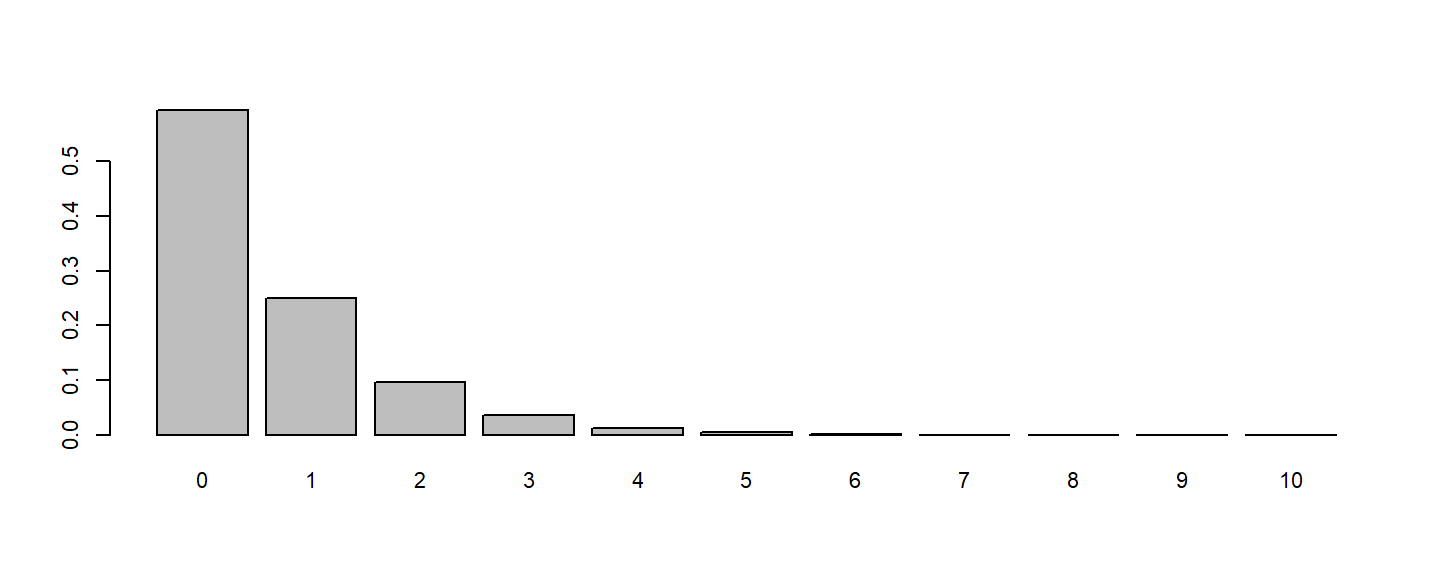💡 Theoretical Discrete Distributions

• Binomial[n, p]: the distribution of the number of successes, while repeating an experiment of success rate p for n times.
• Geometric[p]: the distribution of the number of failures before the first success, while repeating an experiment of success rate p.
• NBinomial[n, p]: the distribution of the number of failures before the n-th success, while repeating an experiment of success rate p.
• Poisson[$$\lambda$$]: the distribution of the number of successes given the expected value $$\lambda$$.

pacman::p_load(dplyr, vcd)

### 【1】Death by Horse Kick

HorseKick: Number of events per year per corps

par(mfrow=c(1,1), cex=0.7)
HorseKicks
nDeaths
0   1   2   3   4
109  65  22   3   1 

Test H0: The data fits Poisson Distribution

fit = goodfit(HorseKicks, type = "poisson")
summary(fit)

Goodness-of-fit test for poisson distribution

X^2 df P(> X^2)
Likelihood Ratio 0.86822  3  0.83309

p=0.833 > 0.05：The data is not significantly different from Poisson.

Parameters：What is the $$\lambda$$?

fit$par $lambda
 0.61

Application：What is the probability of nDeath >= 2

1 - ppois(1, fit$par$lambda)  
 0.12521

🧙 Discussion：
If an insurance company want to design an policy for death by horse kick, and you need to know P[nDeath>5]：
■ Can you estimate the Probability from the data？
■ Can you estimate it by the model？
■ Which way is better？

What is the probability of nDeath >= 5?

1 - ppois(4, fit$par$lambda)  
 0.00042497

### 【2】“May” in Federalist Papers

Data Federalist：In an set of Federal Papers, the numbers of “may” per paragraph.

Federalist
nMay
0   1   2   3   4   5   6
156  63  29   8   4   1   1 

TestH0: The data fits Poisson Distribution

fit <- goodfit(Federalist, type = "poisson")
summary(fit)

Goodness-of-fit test for poisson distribution

X^2 df   P(> X^2)
Likelihood Ratio 25.243  5 0.00012505
• It significantly differs from Poisson

H0: The data fits Negative Binomial Distribution

fit = goodfit(Federalist, type = "nbinomial")
summary(fit)

Goodness-of-fit test for nbinomial distribution

X^2 df P(> X^2)
Likelihood Ratio 1.964  4  0.74238
• It does not significantly differs from Negative Binomial

Parameters：What are the parameters？

fit$par $size
 1.1863

$prob  0.64376 • It complies to NBinom[n=1.19, p=0.64]. Plot：How does the distribution looks like? par=c(margin=c(3,3,3,1),cex=0.7) dnbinom(0:10, fit$par$size, fit$par\$prob) %>% barplot(names=0:10)Estimation：What is the probability that 2 <= nMay <= 6?

# 

💡 Steps to Apply Theoretical Distribution

1. Test for fitness
2. Estimate Parameters
3. Estimate Probabilities of Events